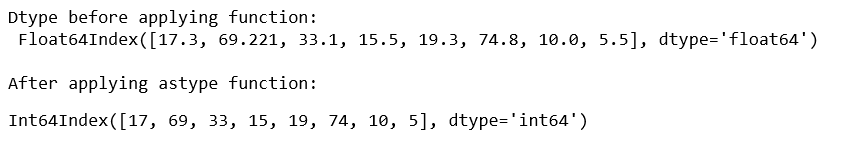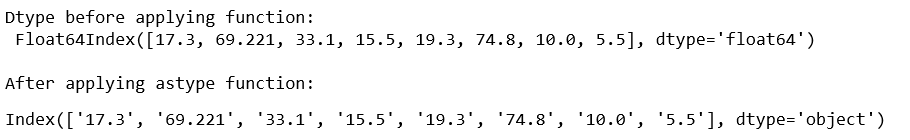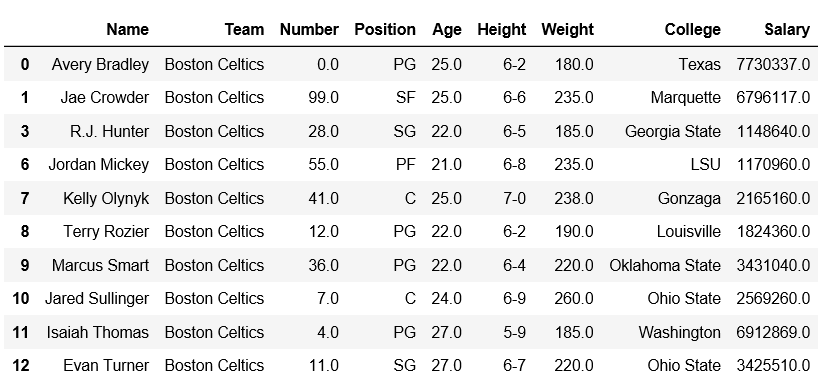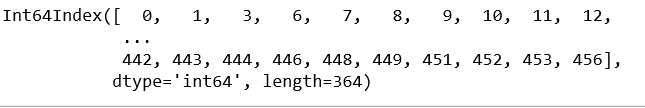Python | Pandas Index.astype()

• Last Updated : 16 Dec, 2018

Python is a great language for doing data analysis, primarily because of the fantastic ecosystem of data-centric python packages. Pandas is one of those packages and makes importing and analyzing data much easier.

Pandas Index.astype() function create an Index with values cast to dtypes. The class of a new Index is determined by dtype. When conversion is impossible, a ValueError exception is raised.

Syntax: Index.astype(dtype, copy=True)

Parameters :
dtype : numpy dtype or pandas type
copy : By default, astype always returns a newly allocated object. If copy is set to False and internal requirements on dtype are satisfied, the original data is used to create a new Index or the original Index is returned.

Example #1: Use Index.astype() function to change the data type of index from float to integer type.

 # importing pandas as pdimport pandas as pd   # Creating the Indexdf=pd.Index([17.3, 69.221, 33.1, 15.5, 19.3, 74.8, 10, 5.5])  print("Dtype before applying function: \n", df)  print("\nAfter applying astype function:")# Convert df datatype to 'int64'df.astype('int64')

Output :Example #2: Use Index.astype() function to change the datatype of the given Index to string form.

 # importing pandas as pdimport pandas as pd   # Creating the Indexdf=pd.Index([17.3, 69.221, 33.1, 15.5, 19.3, 74.8, 10, 5.5])  print("Dtype before applying function: \n", df)  print("\nAfter applying astype function:")# Convert df datatype to 'int64'df.astype('str')

Output :Example #3: Let’s do something interesting with index.astype() method.

Observe this DataFrame.Setting ‘Number’ column as index.

 # importing pandas module  import pandas as pd     # reading csv file from url  data = pd.read_csv("https://media.geeksforgeeks.org/wp-content/uploads/nba.csv")      # dropping null value columns to avoid errors data.dropna(inplace = True)   # Setting Number column as indexdata = data.set_index('Number')  # Setting index as Nonedata.index.names = [None]data.head(5)

Output:Now, let’s convert the index to integer.

 # applying astype on indexdata.index.astype('int64')

Output:My Personal Notes arrow_drop_up